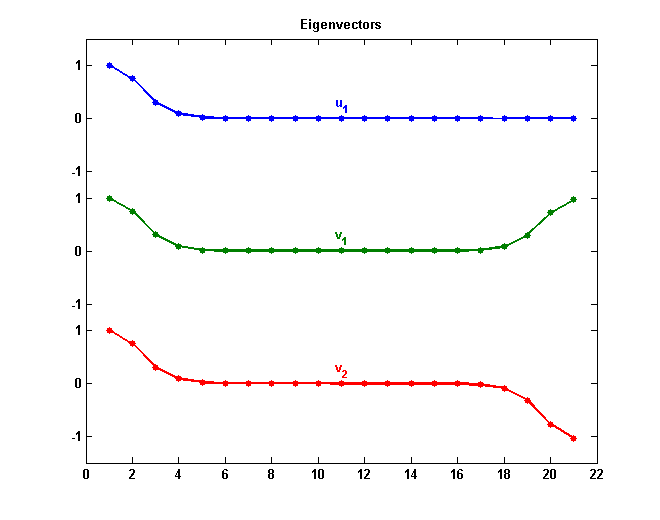# Wilkinson’s Matrices

One of Jim Wilkinson's outstanding skills was his ability to pick great examples. A previous post featured his signature polynomial. This post features his signature matrices.

### Contents

#### Tridiagonal Matrices

When working with eigenvalues, we always have to be concerned about multiplicity and separation. Multiple eigenvalues and clusters of close eigenvalues require careful attention. Symmetric tridiagonal matrices are easier to handle than the general case. If none of the off diagonal elements are zero, there are no multiple eigenvalues. But if none of the off diagonal elements are "small", can we conclude that the eigenvalues are reasonably well separated? One of Wilkinson's matrices answers this question with a resounding "no".

#### Wilkinson's Matrices

Wilkinson introduces the matrices $W_{2n+1}^-$ and $W_{2n+1}^+$ on page 308 of The Algebraic Eigenvalue Problem and then employs them throughout the rest of the book. These matrices are the families of symmetric tridiagonal matrices generated by the following MATLAB function. ($W_n^+$ is also generated by the function wilkinson in toolbox\matlab\elmat that has been part of MATLAB for many years.)

type generate_wilkinson_matrices

function [Wm,Wp] = generate_wilkinson_matrices(n)

D = diag(ones(2*n,1),1);
Wm = diag(-n:n) + D + D';
Wp = abs(diag(-n:n)) + D + D';



Here, for example, is $n = 3, 2n+1 = 7$.

format compact
[Wm,Wp] = generate_wilkinson_matrices(3)

Wm =
-3     1     0     0     0     0     0
1    -2     1     0     0     0     0
0     1    -1     1     0     0     0
0     0     1     0     1     0     0
0     0     0     1     1     1     0
0     0     0     0     1     2     1
0     0     0     0     0     1     3
Wp =
3     1     0     0     0     0     0
1     2     1     0     0     0     0
0     1     1     1     0     0     0
0     0     1     0     1     0     0
0     0     0     1     1     1     0
0     0     0     0     1     2     1
0     0     0     0     0     1     3


#### Eigenvalues

Wilkinson usually has $2n+1 = 21$. Here are the eigenvalues of $W_{21}^-$ and $W_{21}^+$.

format long
[Wm,Wp] = generate_wilkinson_matrices(10);
disp('       eig(Wm(21))         eig(Wp(21))')
disp(flipud([eig(Wm) eig(Wp)]))

       eig(Wm(21))         eig(Wp(21))
10.746194182903357  10.746194182903393
9.210678647333035  10.746194182903322
8.038941119306445   9.210678647361332
7.003952002665359   9.210678647304919
6.000225680185169   8.038941122829023
5.000008158672943   8.038941115814275
4.000000205070442   7.003952209528674
3.000000003808129   7.003951798616375
2.000000000054486   6.000234031584166
1.000000000000619   6.000217522257097
0.000000000000003   5.000244425001915
-1.000000000000618   4.999782477742903
-2.000000000054490   4.004354023440857
-3.000000003808127   3.996048201383625
-4.000000205070437   3.043099292578824
-5.000008158672947   2.961058884185726
-6.000225680185168   2.130209219362506
-7.003952002665363   1.789321352695084
-8.038941119306442   0.947534367529292
-9.210678647333047   0.253805817096678
-10.746194182903357  -1.125441522119985


#### Properties

Here are some important properties of these eigenvalues.

• All the eigenvalues of $W_{2n+1}^-$, except the middle one, occur in $\pm$ pairs.
• $W_{2n+1}^-$ is singular. The middle eigenvalue is zero.
• All the eigenvalues of $W_{2n+1}^+$, but one, are positive.
• The positive eigenvalues of $W_{2n+1}^+$ occur roughly in pairs, with a positive eigenvalue of $W_{2n+1}^-$ approximately in the center of each pair.
• The larger pairs of eigenvalues of $W_{2n+1}^+$ are incredibly close together.

#### Remarkable Closeness

All of the off diagonal elements in $W_{21}^+$ equal one, so none are "small", and yet the first two eigenvalues of $W_{21}^+$ agree to fourteen significant figures. Wilkinson calls this "remarkable" and "pathological". The relative distance is

format short e
e = flipud(eig(Wp));
delta = (e(1)-e(2))/e(1)

delta =
6.6120e-15


#### Eigenvectors

The behavior of the eigenvalues can be understood by looking at the eigenvectors. Here is the vector $u_1$ associated with the first eigenvalue of $W_{21}^-$ and the vectors $v_1$ and $v_2$ associated with the leading pair of eigenvalues of $W_{21}^+$, Each vector has been normalized so that its first component is one.

[U,~] = eig(Wm);
[V,~] = eig(Wp);
u1 = flipud(U(:,end)/U(end,end));
v1 = flipud(V(:,end)/V(end,end));
v2 = flipud(V(:,end-1)/V(end,end-1));

format long
[u1 v1 v2]

ans =
1.000000000000000   1.000000000000000   1.000000000000000
0.746194182903358   0.746194182903392   0.746194182903321
0.302999941502167   0.302999941502254   0.302999941502075
0.085902493869951   0.085902493870164   0.085902493869724
0.018807481330335   0.018807481331049   0.018807481329571
0.003361464615150   0.003361464618322   0.003361464611748
0.000508147087273   0.000508147104788   0.000508147068490
0.000066594309069   0.000066594424058   0.000066594185758
0.000007705362252   0.000007706235465   0.000007704425844
0.000000798285440   0.000000805807738   0.000000790218740
0.000000074882661   0.000000147323229  -0.000000002800555
0.000000006418173   0.000000777356287  -0.000000820314052
0.000000000506441   0.000007428942095  -0.000007992139484
0.000000000037026   0.000064197613845  -0.000069080489810
0.000000000002522   0.000489858240829  -0.000527118748833
0.000000000000161   0.003240481200881  -0.003486964947267
0.000000000000010   0.018130575985481  -0.019509658947141
0.000000000000001   0.082810753074099  -0.089109664858083
0.000000000000000   0.292094585462557  -0.314312449184672
0.000000000000000   0.719337698380754  -0.774053354706959
0.000000000000000   0.964008718993031  -1.037335016061421


And here is a plot of these three eigenvectors. The components of the first half of each of the three vectors decay rapidly and all three agree with each other to many decimal places.

n = 10;
plot_wilkinson_eigenvectorsFor a tridiagonal matrix $A$, the eigenvalue/vector equation

$$Ax = \lambda x$$

is a three-term recurrence relation for the components of $x$. For the first half of each of the three vectors, we have the same positive elements on the diagonal of $A$, ones on the off diagonal, and nearly the same positive value of $\lambda$, so the recurrence relation is nearly the same and leads to nearly the same rapidly decreasing components.

Once the recurrence relation gets half way through the vector and the components get small, this argument breaks down. For $u_1$ the diagonal elements are negative and so the components of the vector remain small. Perron-Frobenius implies that $v_1$ goes positive. Symmetry implies that $v_2$ goes negative to stay orthogonal to $v_1$.

#### Perturbation Theory

A closer examination of the elements of $v_1$ reveals that they decay exponentially until they reach a minimum half way through the vector at index $n+1$. It is possible to estimate a priori that this minimum value is of order $O(1/n!)$

format short e
minimum = v1(n+1)
estimate = 1/factorial(n)

minimum =
1.4732e-07
estimate =
2.7557e-07


This information about the shape of the dominant eigenvector makes it possible to prove that the corresponding eigenvalue, $\lambda_1$, is a double eigenvalue with separation of order the square of the minimum in the eigenvector, which is $O(1/(n!)^2)$.

separation = e(1)-e(2)
estimate = 1/factorial(n)^2

separation =
7.1054e-14
estimate =
7.5941e-14


#### Acknowledgement

Thanks to Pete Stewart, University of Maryland, for some helpful email while I was working on this post.

#### Reference

J. H. Wilkinson, The Algebraic Eigenvalue Problem, Claredon Press, Oxford, 1965, 662 pp.

Published with MATLAB® R2012b

|